# Recursive Bayesian Filtering

## Kalman Filter

### Assumed model

We assume the state to be random variable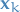as follows: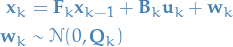1

and the measurements or observations to be a random variable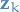: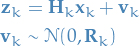2

I.e. both stateand measurementare assumed to be normally distributed with covariance-matrices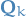and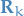, respectively.

### Deduction

#### Notation

•state
•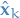predicted state
•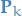covariance matrix for the predicted state
•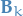control-matrix, e.g. mapping external forces to how it affects the state
•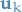control-vector, e.g. external force
•covariance matrix describing external uncertainty from the environment
•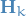maps the predicted state to the measurement-space, i.e. "predict" measurement from state
•real measurement
•uncertainty in real measurements

#### Algorithm

We start out by writing the predicted stateas a function of the previous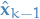predicted state: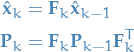3

Whereis due to the following identify: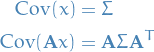4

I.e. mapping the covariance at step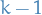to the covariance of the state at step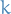.

The next step is then to incorporate "external forces", where we use:

•- control vector which defines how
•- the matrix

I like to view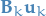as a simple function mapping the control-vectorto the state-space, or rather, a function which maps the external "force" to how this "force" affects the state.

This, together with the uncertainty of the external "forces" / influences: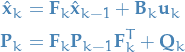5

Now, what if we can see the real measurement for step,, before proceeding? Well, we would like to update our model! To do this, we need to relate the predicted state to the real state . We thus write the predicted measurement as: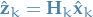6

Now, we can then write the real measurement as follows: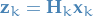7

And we of course want the following to be true: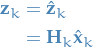8

Therefore we can map the predicted state to the measurement-space by using the matrix.

Thus we have a model for making the predictions, now we need to figure out how to update our model!

For this we first map our "internal" stateto the measurement-space. In this case, our measurements only consists of the position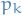.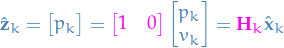Thus, the predicted measurements follow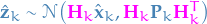and the real measurements follow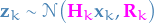, whereis the "real" internal state.

To compute our updated state, we can then take the joint distribution of the predicted and real measurements, incorporating information from both our estimate and the current measurement.

Due to the joint-probability of two Gaussian rvs. also being Guassian, with mean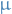and covariance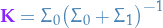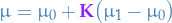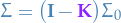In the context of Kalman filters we call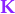the Kalman gain.

By letting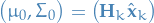and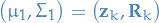, we get the update-equations: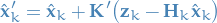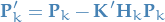where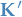iswithout thein front.

### Summary

Predict!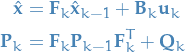9

Update! Since our predictive model is Gaussian, and we assume a Gaussian distribution for our measurements, we simply update our model parameters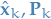by taking the joint-distribution of these two Gaussian rvs., which again gives us a Guassian distribution with the new updated paramters: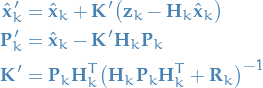10

#### Prediction11

#### Update

Since our predictive model is Gaussian, and we assume a Gaussian distribution for our measurements, we simply update our model parametersby taking the joint-distribution of these two Gaussian rvs., which again gives us a Guassian distribution with the new updated parameters:12

## Particle Filtering

### Notation

•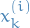denotes the k-th sampled step of the i-th "particle" / sample-sequence
•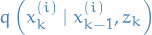is the "importance distribution" which we can sample from

### Overview

• Can represent arbitrary distributions
• But this is an approximate method, unlike method such as Kalman Filter which are (asymptotically) exact and "optimal"
• Name comes from the fact that we're keeping track of multiple "particles"
• "Particle" is just a single "chain" of samples
• Main building-block is Importance Sampling (IS)
• "Extended" to sequences, which we call Sequential Importance Sampling

### Sequential Importance Sampling (SIS)

Sequential Importance Sampling (SIS) extends Importance Sampling (IS) by updating the sample-weights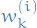sequentially.

The particle and weight updates, respectively, are defined by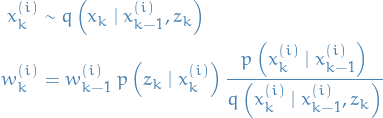IMPORTANT: normalize the weights after each weight-update, such that we can use the weights to approximate the expectations.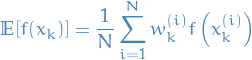### Degeneracy phenomenon

• Usually after a few iterations of SIS most particles have neglible weights
• => large computation efforts for updating particles with very small contributions: highly inefficient
• One can "measure" degeneracy using the "effective sample size":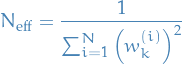• Uniform: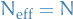• Severe degeneracy: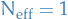• To deal with degeneracy we introduce resampling
1. When degeneracy is above a threshold, eliminate particles with low importance weights
2. Sample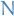new samples / "particles"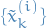by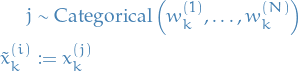i.e. we're generating new particles by drawing from the current particles, each with probability equal to the corresponding weight.

• If we eliminiated the particles with low importance weights, the corresponding weights should of course not be included.
3. New samples and weights are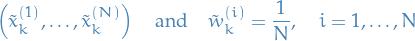i.e. new weights are uniform.

A good threshold is a "measure" of degeneracy using the "effective sample size":• Uniform:• Severe degeneracy:#### Practical considerations

Implementing the categorical sampling method used in resampling can efficiently be performed by

1. Normalize weights
2. Calculate an array of the cumulative sum of the weights
• Partitions the range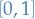intointervals
• If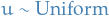, then the interval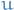falls into is the corresponding3. Randomly generateuniform numbers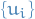and determine the corresponding samples

### Generic Particle Filtering

Combining SIS and resampling, we get generic Particle Filtering.

At each "step", we "obtain new states" / "update the particles" through:

1. Produce tuple of samples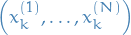using SIS
2. Compute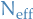• If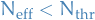: resample!